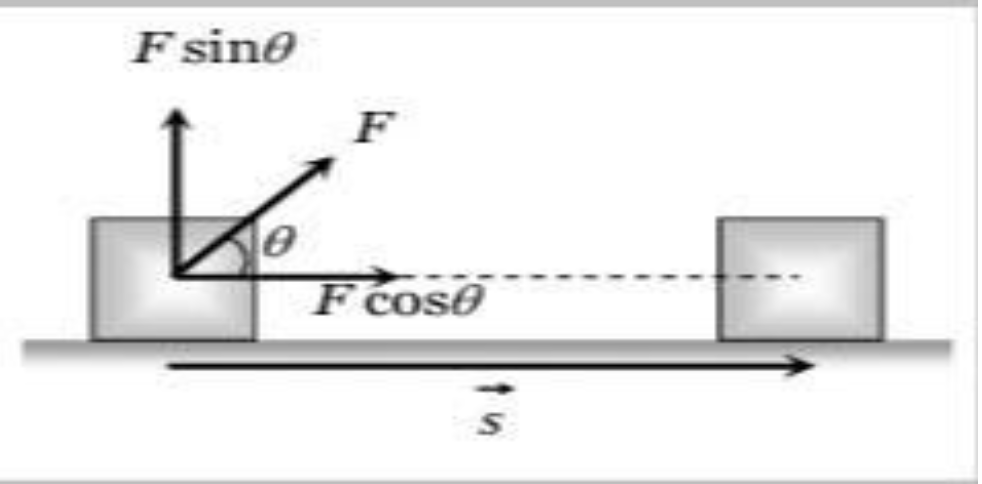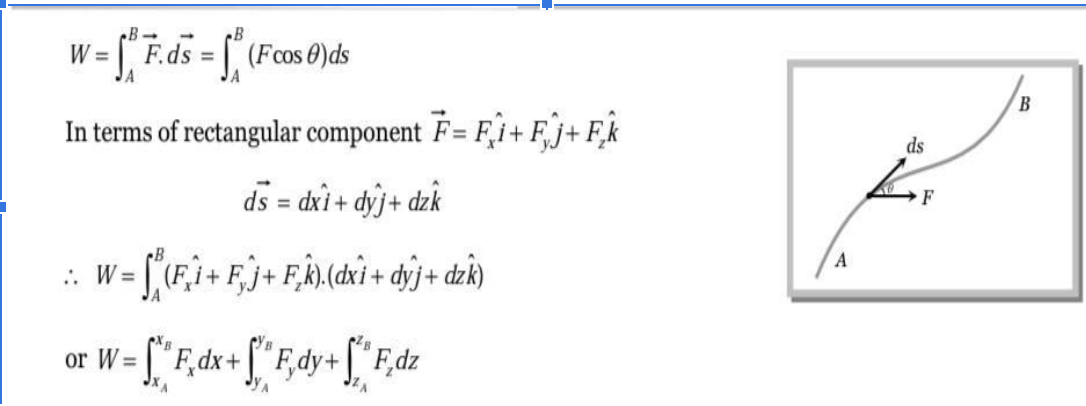# Work , Energy and Power

Work , Energy and Power

Introductuon:

The terms 'work', 'energy' and 'power' are frequently used in everyday language. A farmer clearing weeds in his

field is said to be working hard. A woman carrying water from a well to her house is said to be working. In a drought

affected region she may be required to carry it over large distances. If she can do so, she is said to have a large stamina

or energy. Energy is thus the capacity to do work. The term power is usually associated with speed. In karate, a powerful

punch is one delivered at great speed. In physics we shall define these terms very precisely. We shall find that there is at

best a loose correlation between the physical definitions and the physiological pictures these terms generate in our

minds.  Work is said to be done when a force applied on the body displaces the body through a certain distance in the

direction of force.

Work done by a constant force:

Let a constant force F be applied on the body such that it makes an angle θ with the horizontal and body is displaced

through a distance s  By resolving force F into two components :

(i) F cos θ in the direction of displacement of the body.

(ii) F sin θ in the perpendicular direction of displacement of the body.  Since body is being displaced in the direction of F cos θ, therefore work done by the force in displacing the body through a distance s is given byW= F.cos θ

Thus work done by a force is equal to the scalar or dot product of the force and the displacement of the body. If a number of force F1+F2+F3+………

Work Done by a Variable Force:

When the magnitude and direction of a force varies with position, the work done by such a force for an infinitesimal displacement is given by  dW=F.ds The total work done in going from A to B as shown in the figure is• Dimensions and unit of Work : W= Force x displacement
• ML^2T^-2
• The unit of work has two types.